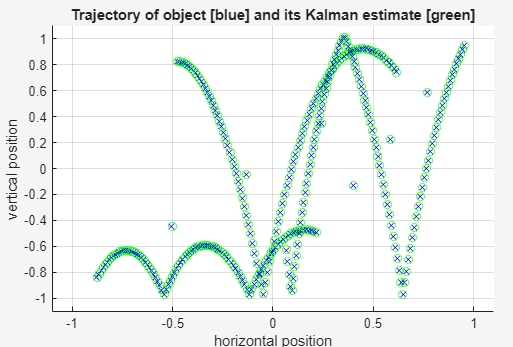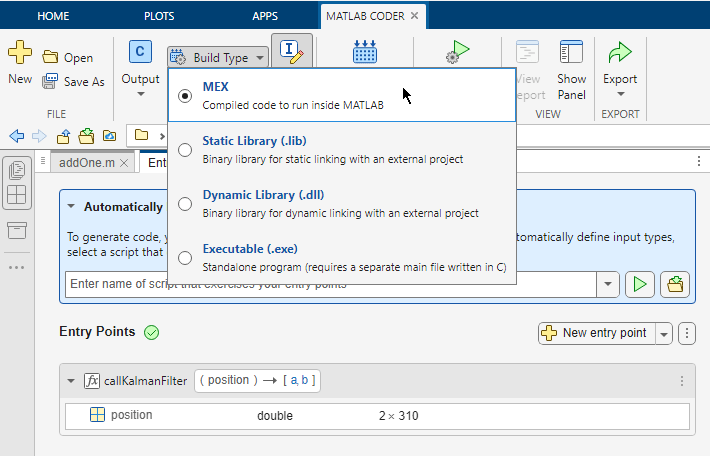# Unit Test External C Code with MATLAB Coder

This example shows how to test external C code by using MATLAB® unit tests with MATLAB® Coder™.

If you want to test C code, you can use MATLAB Coder to bring the code into MATLAB. You can then write unit tests by using the MATLAB testing framework. You can write richer, more flexible tests by taking advantage of the advanced numerical computing and visualization capabilities of MATLAB.

This example shows how to:

1. Bring your C code into MATLAB as a MEX function that you generate with MATLAB Coder.

2. Write a unit test by using the MATLAB testing framework.

3. Run the test on the MEX function.

If you have Embedded Coder®, you can run unit tests on generated standalone code (static library or shared library) by using the unit tests with software-in-the-loop (SIL) execution or processor-in-the-loop (PIL) execution.

### Examine the Files

To access the files that this example uses, click Open Script.

kalmanfilter.c

`kalmanfilter.c` is the C function that the example tests. It estimates the position of a moving object based on its past positions.

kalmanfilter.h

`kalmanfilter.h` is the header file for `kalmanfilter.c`.

position.mat

`position.mat` contains the positions of the object.

callKalmanFilter.m

`callKalmanFilter` calls `kalmanfilter` by using `coder.ceval`.

```function [a,b] = callKalmanFilter(position) % Copyright 2014 - 2016 The MathWorks, Inc. numPts = size(position,2); a = zeros(2,numPts,'double'); b = zeros(2,numPts,'double'); y = zeros(2,1,'double'); % Main loop for idx = 1: numPts z = position(:,idx); % Get the input data % Call the initialize function coder.ceval('kalmanfilter_initialize'); % Call the C function coder.ceval('kalmanfilter',z,coder.ref(y)); % Call the terminate function coder.ceval('kalmanfilter_terminate'); a(:,idx) = [z(1); z(2)]; b(:,idx) = [y(1); y(2)]; end end ```

TestKalmanFilter.m

`TestKalmanFilter` tests whether the error between the predicted position and actual position exceeds the specified tolerance. The unit tests are class-based unit tests. For more information, see Author Class-Based Unit Tests in MATLAB.

Although you want to test the MEX function, the unit tests in `TestKalmanFilter` call the original MATLAB function from which you generated the MEX function. When MATLAB Coder runs the tests, it replaces the calls to the MATLAB function with calls to the MEX function. You cannot run these tests directly in MATLAB because MATLAB does not recognize the `coder.ceval` calls in `callKalmanFilter`.

```classdef TestKalmanFilter < matlab.unittest.TestCase % Copyright 2014 - 2016 The MathWorks, Inc. methods ( Test ) function SSE_LessThanTolerance( testCase ) load position.mat; [z,y] = callKalmanFilter( position ); tolerance = 0.001; % tolerance of 0.0001 will break A = z-1000*y; error = sum(sum(A.^2)); testCase.verifyLessThanOrEqual( error, tolerance); % For debugging plot_kalman_filter_trajectory(z,1000*y); end function SampleErrorLessThanTolerance( testCase ) load position.mat; [z,y] = callKalmanFilter( position ); tolerance = 0.01; % tolerance of 0.001 will break A = z-1000*y; testCase.verifyEqual(1000*y, z, 'AbsTol', tolerance); % For debugging plot_kalman_filter_trajectory(z,1000*y); [value, location] = max(A(:)); [R,C] = ind2sub(size(A),location); disp(['Max value ' num2str(value) ' is located at [' num2str(R) ',' num2str(C) ']']); end end end ```

run_unit_tests_kalman.m

`run_unit_tests_kalman` calls `runtests` to run the tests in `TestKalmanFilter.m`.

```% Run unit tests % Copyright 2014 - 2016 The MathWorks, Inc. runtests('TestKalmanFilter') ```

plot_kalman_filter_trajectory.m

`plot_kalman_filter_trajectory` plots the trajectory of the estimated and actual positions of the object. Each unit test calls this function.

### Generate MEX and Run Unit Tests in the MATLAB Coder App

To open the MATLAB Coder app, on the MATLAB Toolstrip Apps tab, under Code Generation, click the MATLAB Coder app icon.

To prepare for code generation, advance through the app steps.

• On the Select Source Files page, specify that the entry-point function is `callKalmanFilter`.

• On the Define Input Types page, specify that the input argument `x` is a 2-by-310 array of doubles.

The unit tests load the variable `position` from `position.mat` and pass `position` to `callKalmanFilter`. Therefore, the input to `callKalmanFilter` must have the properties that `position` has. In the MATLAB workspace, if you load `position.mat`, you see that `position` is a 2-by-310 array of doubles.

• Skip the Check for Run-Time Issues step for this example.

Configure the app for MEX code generation. Specify the names of the C source and header files because `callKalmanFilter` integrates external C code.

1. For Build type, specify `MEX`.

2. Click More Settings.

3. On the Custom Code tab:

• Under Custom C Code for Generated Files, select Header file. In the custom code field, enter `#include "kalmanfilter.h"`.

• In the Additional source files field, enter `kalmanfilter.c`.To generate the MEX function, click Generate.

Run the unit tests on the generated MEX.

1. Click Verify Code.

2. In the field for the test file, specify `run_unit_tests_kalman`.

3. Make sure that you set Run using to Generated code.

4. Click Run Generated Code.When the app runs the test file, it replaces calls to `callKalmanFilter` in the unit test with calls to `callKalmanFilter_mex`. The unit tests run on the MEX function instead of the original MATLAB function.

The app displays the test output on the Test Output tab. The unit tests pass.From the plots, you can see that the trajectory of the estimated position converges with the trajectory of the actual position.### Run Unit Tests After Modifying C Code

When you modify the C code, to run the unit tests:

1. Regenerate the MEX function for the MATLAB function that calls the C code.

2. Repeat the verification step.

For example, modify `kalmanfilter.c` so that the value assigned to `y[r2]` is multiplied by 1.1.

`y[r2] += (double)d_a[r2 + (i0 << 1)] * x_est[i0] * 1.1;`

Edit `kalmanfilter.c` outside of the app because you can use the app to edit only MATLAB files listed in the Source Code pane of the app.

To generate the MEX function for the modified function, click Generate.

To run the unit tests:

1. Click Verify Code.

2. Make sure that you set the test file to `run_unit_tests` and Run using to Generated code

3. Click Run Generated Code.

The tests fail because the error exceeds the specified tolerance.

The plots show the error between the trajectory for the estimated position and the trajectory for the actual position.### Generate MEX and Run Unit Tests by Using the Command-Line Workflow

You can use the command-line workflow to run unit tests on external C code by using `coder.runTest`. Specify a test file that runs the unit tests on the MATLAB function that calls your C code.

Generate a MEX function for the MATLAB function that calls your C code. For this example, generate MEX for `callKalmanFilter`.

Create a configuration object for MEX code generation.

```cfg = coder.config('mex'); ```

Specify the external source code and header file.

```cfg.CustomSource = 'kalmanfilter.c'; cfg.CustomHeaderCode = '#include "kalmanfilter.h"'; ```

To determine the type for the input to `callKalmanFilter`, load the position file.

```load position.mat ```

To generate the MEX function, run `codegen`. Specify that the input to `callKalmanFilter` has the same type as `position`.

```codegen -config cfg callKalmanFilter -args position ```

Run the units tests on the MEX function. Specify that the test file is `run_unit_tests_kalman` and that the function is `callKalmanfilter`. When `coder.runTest` runs the test file, it replaces calls to `callKalmanFilter` in the unit test with calls to `callKalmanFilter_mex`. The unit tests run on the MEX function instead of the original MATLAB function.

```coder.runTest('run_unit_tests_kalman', 'callKalmanFilter') ```
```Running TestKalmanFilter Current plot held .Current plot held Max value 0.0010113 is located at [2,273] . Done TestKalmanFilter __________ ans = 1x2 TestResult array with properties: Name Passed Failed Incomplete Duration Details Totals: 2 Passed, 0 Failed, 0 Incomplete. 25.7985 seconds testing time. ```